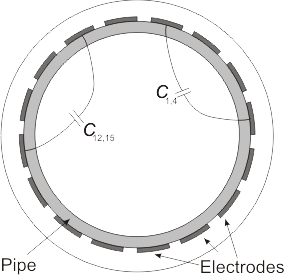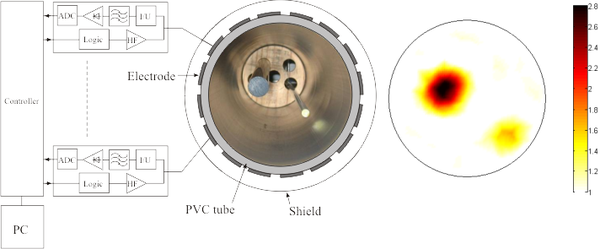# What is Electrical Capacitance Tomography (ECT)?

Electrical Capacitance Tomography (ECT) is a non-invasive method used to obtain the spatial permittivity distribution within Regions of Interest (ROI), which often refers to the interior of closed pipes. The principle is based on the measurement of the capacitances between electrodes located on the exterior of the region of interest, e.g. the of a pipe. In the figure below, two capacitances (C12,15 and C1,4) are shown examplarily.Figure 1: Principle of the ECT measurement system: Electrical Capacitances between a number of electrodes (around the region of interest (ROI)) are measured. The permittivity distribution inside the ROI is reconstructed using this measurement data and sophistacted algorithms.

Since the capacitance depends on the permittivity value of the material located between the electrodes, substances of differing dielectric properties can be distinguished by means of this method. To obtain a spatially resolved image of the permittivity distribution inside the pipe, multiple electrodes are arranged on the tube and all inter-electrode capacitances are measured.

The application of suitable algorithms yields the permittivity distribution inside the pipe. Common ECT systems are based on a linear relationship between capacitance and permittivity values. Such systems, e. g. Linear Back Projection (LBP) or Simultaneous Iterative Reconstruction Techniques (SIRT) systems) are fast but the reconstruction quality is not satisfying since the true relationship between capacitance and permittivity is strongly nonlinear and cannot be approximated by a linear mapping for high-quality reconstruction purposes. Research at the Institute concentrates on the implementation of nonlinear reconstruction principles in order to increase the quality of reconstructed permittivity images and to fulfill the demand on real-time process tomography. Furthermore, with increasing measurement carrier frequencies, wave propagation effects are of intererst. Another important issue are consideration of 3D effects in 2D reconstruction algorithms, in order to obtain reasonably fast reconstruction paired with reasonable image quality.Figure 2: Principle of a ECT Measurement system and example reconstruction result. The shown system  uses 16 electrodes in a circulate arrangement around a PVC pipe with a diameter of  110 mm. The excitation frequency for this sensor amounts to 40 MHz and the frame rate to 100 Hz.

Contact